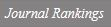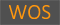EXISTENCE OF WEAK SOLUTIONS TO A CLASS OF SCHRODINGER TYPE EQUATIONS INVOLVING THE FRACTIONAL p-LAPLACIAN IN R-N

Title
EXISTENCE OF WEAK SOLUTIONS TO A CLASS OF SCHRODINGER TYPE EQUATIONS INVOLVING THE FRACTIONAL p-LAPLACIAN IN R-N
Authors
Kim, Jae-MyoungKim, Yun-HoLee, Jongrak
Ewha Authors
Issue Date
2019
Journal Title
JOURNAL OF THE KOREAN MATHEMATICAL SOCIETY
ISSN
0304-99142234-3008Citation
JOURNAL OF THE KOREAN MATHEMATICAL SOCIETY vol. 56, no. 6, pp. 1529 - 1560
Keywords
fractional p-Laplacianvariational methodscritical point theory
Publisher
KOREAN MATHEMATICAL SOC
Indexed
SCIE; SCOPUS; KCIDocument Type
Article
Abstract
We are concerned with the following elliptic equations: (-Delta)(p)(s)u + V(x)vertical bar u vertical bar(p-2)u = lambda g(x,u) in R-N, where (-Delta)(p)(s) is the fractional p-Laplacian operator with 0 < s < 1 < p < + infinity, sp < N, the potential function V : R-N -> (0, infinity) is a continuous potential function, and g : R-N x R -> R satisfies a Caratheodory condition. We show the existence of at least one weak solution for the problem above without the Ambrosetti and Rabinowitz condition. Moreover, we give a positive interval of the parameter lambda for which the problem admits at least one nontrivial weak solution when the nonlinearity g has the subcritical growth condition.
DOI
10.4134/JKMS.j180785
Appears in Collections:
연구기관 > 수리과학연구소 > Journal papers
Files in This Item:
There are no files associated with this item.
Export
RIS (EndNote)
XLS (Excel)
XML